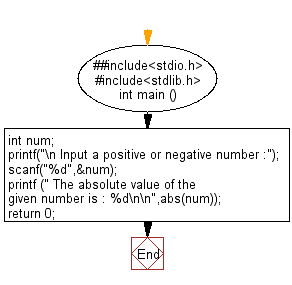﻿ C Program: Return the absolute value of an integer - w3resource

# C Exercises: Return the absolute value of an integer

## C Variable Type : Exercise-16 with Solution

Write a C program to return the absolute value of an integer.

Sample Solution:

C Code:

``````#include<stdio.h>      // Include the standard input/output header file.
#include<stdlib.h>     // Include the standard library header file.

int main ()          // Start of the main function.
{
int num;          // Declare an integer variable 'num'.

printf("\n Input a positive or negative number :");   // Prompt the user to input a number.
scanf("%d",&num);   // Read the user's input and store it in 'num'.

printf (" The absolute value of the given number is : %d\n\n",abs(num));   // Calculate and print the absolute value of 'num'.

return 0;   // Return 0 to indicate successful execution of the program.
}   // End of the main function.
```
```

Sample Output:

``` Input a positive or negative number :-25
The absolute value of the given number is : 25
```

Flowchart:What is the difficulty level of this exercise?

Test your Programming skills with w3resource's quiz.

﻿